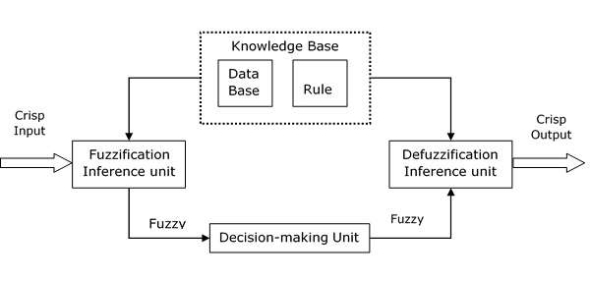# Fuzzy Inference Systems/Mamdani's Methods/Fuzzy Logic Toolbox Quiz

10 Questions | Attempts: 9898SettingsThis is a Fuzzy Ready Here is the very informative Inference Systems/Mamdani's Methods/Fuzzy Logic Toolbox quiz. Fuzzy inference is the process of constructing the mapping from a given input to output using fuzzy logic, which has been applied in various fields such as automatic control, data classification, decision analysis, expert systems, and computer vision. It is associated with a number of names such as fuzzy-rule-based systems, fuzzy expert systems, fuzzy modeling, fuzzy associative memory, fuzzy logic controllers. Try out the quiz and further enhance our knowledge. All the best!

• 1.
What Is Fuzzy Inference Systems?
• A.

The process of formulating the mapping from a given input to an output using fuzzy logic

• B.

Changing the output value to match the input value to give it an equal balance

• C.

Having a larger output than the input

• D.

Having a smaller output than the input

• 2.
What Are The Two Types Of Fuzzy Inference Systems?
• A.

Model-Type and System-Type

• B.

Momfred-Type and Semigi-Type

• C.

Mamdani-Type and Sugeno-Type

• D.

Mihni-Type and Sujgani-Type

• 3.
Where Has Fuzzy Inference Systems Been Implemented?
• A.

Wireless services, heat control, and printers.

• B.

Restrict power usage, telephone lines, and sort data.

• C.

• D.

Automatic control, decision analysis, and data classification.

• 4.
What Is Another Name For Fuzzy Inference Systems?
• A.

Fuzzy Expert System

• B.

Fuzzy Modelling

• C.

Fuzzy Logic Controller

• D.

All of the above

• 5.
Mamdani's Fuzzy Inference Method Was Designed To Attempt What?
• A.

Control any two combinations of any two products by synthesizing a set of linguistic control rules obtained from experienced human operations.

• B.

Control a television and remote combination by synthesizing a set of linguistic control rules obtained from experienced human operations.

• C.

Control a steam engine and a boiler combination by synthesizing a set of linguistic control rules obtained from experienced human operations.

• D.

Control an air craft and fuel level combination by synthesizing a set of linguistic control rules obtained from experienced human operations.

• 6.
What Is The First Step Of the Fuzzy Logic Toolbox?
• A.

Fuzzification of the input variables

• B.

Defuzzification

• C.

Application of the fuzzy operator (AND or OR) in the antecedent

• D.

Aggregation of the consequents across the rules

• 7.
What Is The Input And Output Of Step 2 - Apply Fuzzy Operator?
• A.

The input is a single truth value, and the output has two or more values.

• B.

The input is a value greater than one, and the output is a value less than the input.

• C.

The input and output have both the same values.

• D.

The input has two or more values, and the output has a single truth value.

• 8.
What Is The Equation For Probabilistic?
• A.

Probor (a,b) = a-b + ab

• B.

Probor (a,b) = ab + ab

• C.

Probor (a,b) = a+b - ab

• D.

Probor (a,b) = a/b x ab

• 9.
What Is The Input And Output Of Step 3 - Apply Implication Method?
• A.

Input is a fuzzy set, but the output is a whole value.

• B.

Input is a whole value, but the output can be a fuzzy set.

• C.

Input and output have the same value.

• D.

Input is a smaller value than the output value.

• 10.
What Is The Purpose Of Aggregation?
• A.

To gather all the different fuzzy set outputs and combine them into a single fuzzy set outputs.

• B.

To gather all the possible inputs and use the average to gain an output.

• C.

To gather all the different fuzzy set outputs and average them out to get a single value.

• D.

To subtract all the output fuzzy set values from the input values.

## Related TopicsBack to top
×

Wait!
Here's an interesting quiz for you.